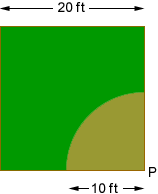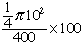Name: Brad Who is asking: Student Level: Secondary Question: I have a problem I can't get. The problem is: A goat is tied to one corner of a square pen 20 ft. on a side. If the goat's tether is 10 ft. long, what percentage of the grass in the pen can the goat reach? What should the length of the tether be so that the goat can reach half of the grass? Thanks! Hi Brad, I drew a diagram. The goat is tethered to a post at the corner labeled P.The area of the pen is 200 x 200 = 400 sq ft. The region where the goat can graze is one-quarter of a circle of radiu 10 ft. The area of a circle is pi r2 and hence the area of the region where the goat can graze is  1/4 pi 102 sq ft. Thus the percentage of the grass in the pen that the goat can reach iswhich is approximately 20%. Now try the second part of the problem. Penny Go to Math Central